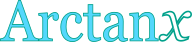Inverse tangent calculator

This tool evaluates the inverse tangent of a number: arctan(x). The principal branch is evaluated, where the return values range between -π/2 and π/2.

 x = Result: arctan(x) = deg radDefinitions

General

The inverse tangent function, in modern notation written as arctan(x), gives the angle θ, so that:

Due to the periodical nature of the tangent function, there are many angles θ that can give the same tangent value (i.e. θ+π, θ+3π, etc.). As a result, it is impossible to define a single inverse function, unless the range of the return values is restricted, so that a one-to-one relationship between θ and tanθ can be established. Therefore, multiple branches of the arctan function can be defined. Commonly, the desired range of θ values spans between -π/2 and π/2. The branch of arctan, in that case, is called the principal branch.

Series

The arctan function can be defined in a Taylor series form, like this:

From the expanded form of the series, it can be seen that the higher terms become insignificant, for values of x close to zero, resulting in the following quite useful approximation: